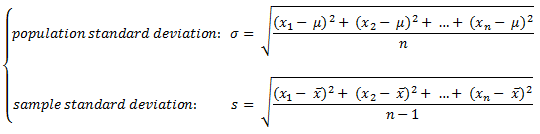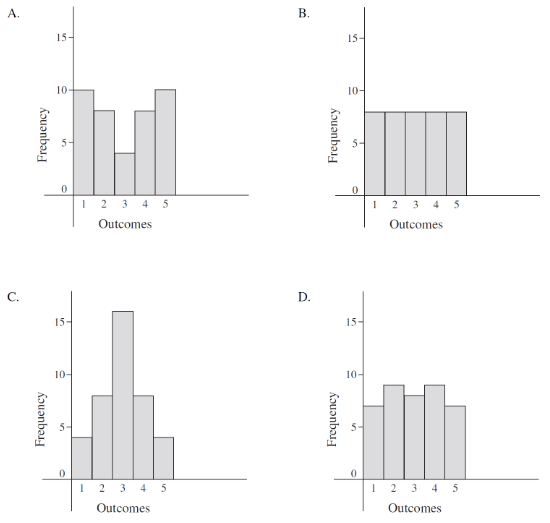# Spread of a data set - standard deviation & variance

## Standard deviation vs variance

In this lesson, we'll be learning how to find standard deviation and variance.

Standard Deviation tells us how spread out a set of numbers (a population) are. In statistics, the population standard deviation is represented by the Greek letter sigma: $\sigma$.

The formula to finding $\sigma$ is as follows:

Population Standard Deviation = $\sqrt{\frac{(x_{1} - \mu)^{2}+...(x_{n} - \mu)^{2}}{n}}$

To explain this formula, remember that you'll need to go through 3 steps when you're looking for the standard deviation.

1) Find the mean of the population

2) For each number in the population subtract it from the mean then square the answer (squared differences)

3) Calculate the average of the squared differences

4) Take the square root of the answer you get from 3

Variance builds off of the standard deviation. The symbol for a population variance is: $\sigma ^{2}$.

You'll find that once you find the standard deviation of a question, you can then easily find the variation. We'll be trying this out with you through some guided examples.

## How to calculate standard deviation

Question 1:

The heights of students (in cm) in a class are: {148, 156, 160, 164, 164, 167, 171, 176, 180, 194}. Find the Population Standard Deviation.

Solution:

To find Population Standard Deviation:

Population Standard Deviation = $\sqrt{\frac{(x_{1} - \mu)^{2}+...(x_{n} - \mu)^{2}}{n}}$

$\mu = \sqrt{\frac{148+...+194}{10}} = 168$

$\sqrt{\frac{(148 - 168)^{2}+...}{10}} = \sqrt{153.1} = 12.385$

What we've done in this question is take each number we are given in terms of the different heights of students and subtracted each of them by the mean. Then we square this number. Again, these numbers are known as squared differences. We'll add all the squared differences together and then divide it by how many different numbers there are in our population of data.

We've got 10 different heights, so the squared differences that we've summed up will be divided by 10. Lastly, we'll take the square root of the number to find the final standard deviation of the population.

## How to calculate variance

Question 2:

The heights of students (in cm) in a class are: {148, 156, 160, 164, 164, 167, 171, 176, 180, 194}. Find the Variance.

Solution:

To find Variance:

$\sigma ^{2} = \sqrt{153.1} ^{2} = 153.1$

What we're doing here is we're making use of the idea that the variance is just the standard deviation squared. So if we take the unsimplified version from question 1, we can square the square root, which removes the square root. We then find that the variance equals 153.1.

## How to calculate sample standard deviation

Asides from finding the population standard deviation, you can take a sample from the population and find the sample standard deviation. Its calculation is similar to finding the population standard deviation but the denominator is different. We'll be using this formula instead:

Sample Standard Deviation = $\sqrt{\frac{(x_{1} - \overline{x})^{2}+...(x_{n} - \overline{x})^{2}}{n - 1}}$

You'll notice that the denominator is now (n-1). Think of this as a way to correct your data when you're working with a sample from a population.

Let's try out a question to find out how to do this.

Question 3:

The heights of students (in cm) in a class are: {148, 156, 160, 164, 164, 167, 171, 176, 180, 194}.

Randomly pick 3 data values and calculate the Sample Standard Deviation

Solution:

To find Sample Standard Deviation:

Let's use 148,264,176 as the random samples

Sample Standard Deviation = $\sqrt{\frac{(x_{1} - \overline{x})^{2}+...(x_{n} - \overline{x})^{2}}{n - 1}}$

$\overline{x} = \frac{148+164+176}{3} = 162.\overline{66}$

Sample Standard Deviation = $\sqrt{\frac{(148 - 162.\overline{66})^{2}+(164 - 162.\overline{66})^{2}+(176 - 162.\overline{66})^{2}}{3 - 1}}$

$= \sqrt{197.\overline{33}} \thickapprox 14.05$

## How to find sample variance

This is pretty straightforward. The sample variance can actually then be found by squaring the number you get for the sample standard deviation from above!

If you wanted a reference for double checking your answers for questions relating to variance and standard deviation, here's an online calculator for just that!

### Spread of a data set - standard deviation & variance

#### Lessons

Standard Deviation measures the average distance data values are from the mean.note:
When the values in a data set are tightly bunched together, the standard deviation will be small.
When the values in a data set are spread apart, the standard deviation will be relatively large.

$variance=\sigma^2$
• 1.
Determining Population Standard Deviation VS. Sample Standard Deviation
The heights of students (in cm) in a class are: {148, 156, 160, 164, 164, 167, 171, 176, 180, 194}
a)
Calculate the Population Standard Deviation and Variance.

b)
Randomly pick 3 data values and calculate the Sample Standard Deviation.

c)
Verify the results using statistics commands on your calculator.

• 2.
Determining the Standard Deviations from Histograms
Without calculation, determine which set of data is likely to have:
• the greatest standard deviation
• the smallest standard deviation• 3.
Determining the Mean and the Standard Deviation from a Frequency Table
Determine the mean and the standard deviation for the population of scores in the following frequency table.

 score frequency 4 6 5 3 7 8 9 2